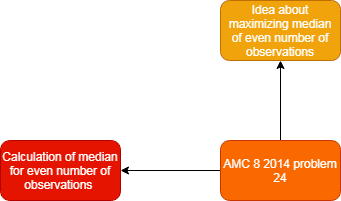Categories

# Calculating the median of observations AMC 8 2014 Problem 24

Try this beautiful problem from AMC 8. It is based on calculating the median of even number of observations. We provide sequential hints so that you can try the problem.

# What are we learning ?

Competency in Focus: Calculating the median of even number of observations  This problem from American Mathematics contest (AMC 8, 2014) is based on maximising median of even number of observations.

# First look at the knowledge graph.# Next understand the problem

One day the Beverage Barn sold$252$ cans of soda to$100$ customers, and every customer bought at least one can of soda. What is the maximum possible median number of cans of soda bought per customer on that day?
##### Source of the problem
American Mathematical Contest 2014, AMC 8 Problem 24
##### Key Competency
Calculation of median for even number of observations .

5/10

##### Suggested Book
Challenges and Thrills in Pre College Mathematics Excursion Of Mathematics

Do you really need a hint? Try it first!
Suppose the numbers of cans purchased by the 100 customers are listed in increasing order.Now median is the average of the 50th and 51th numbers in the ordered list.
Now look -How can you maximise the median ?
In order to maximize the median, we need to make the first half of the numbers as small as possible. To minimise the median ,minimise the first 49 numbers by taking them all to be 1.As given  every customer bought at least one can of soda.
To minimize the first 49, they would each have one can. Subtracting these$49$ cans from the$252$ cans gives us$203$ cans left to divide among$51$ people.If the 50th number is 4,then the sum of all 100 numbers would  at least 49+51.4=253, which is too large .If instead the 50th number is 3 and the following numbers all equal 4 ,then the sum of the 100 numbers is 49+3+50.4=252.Now it’s fine right!
Thus the median is  the average of$3$ and$4$ is$3.5$. Thus our answer is 3.5.

# Connected Program at Cheenta

#### Amc 8 Master class

Cheenta AMC Training Camp consists of live group and one on one classes, 24/7 doubt clearing and continuous problem solving streams.

# Similar Problems

This site uses Akismet to reduce spam. Learn how your comment data is processed.